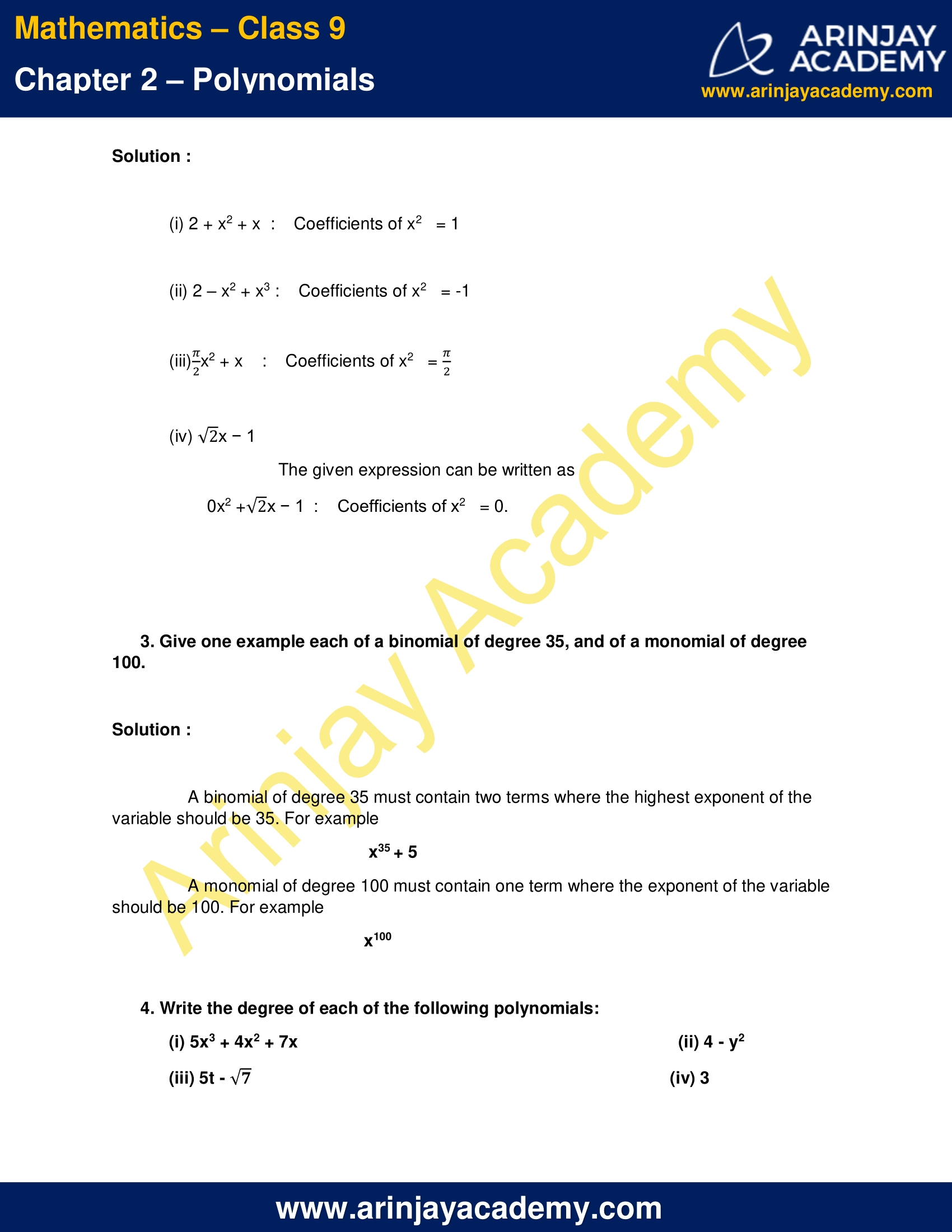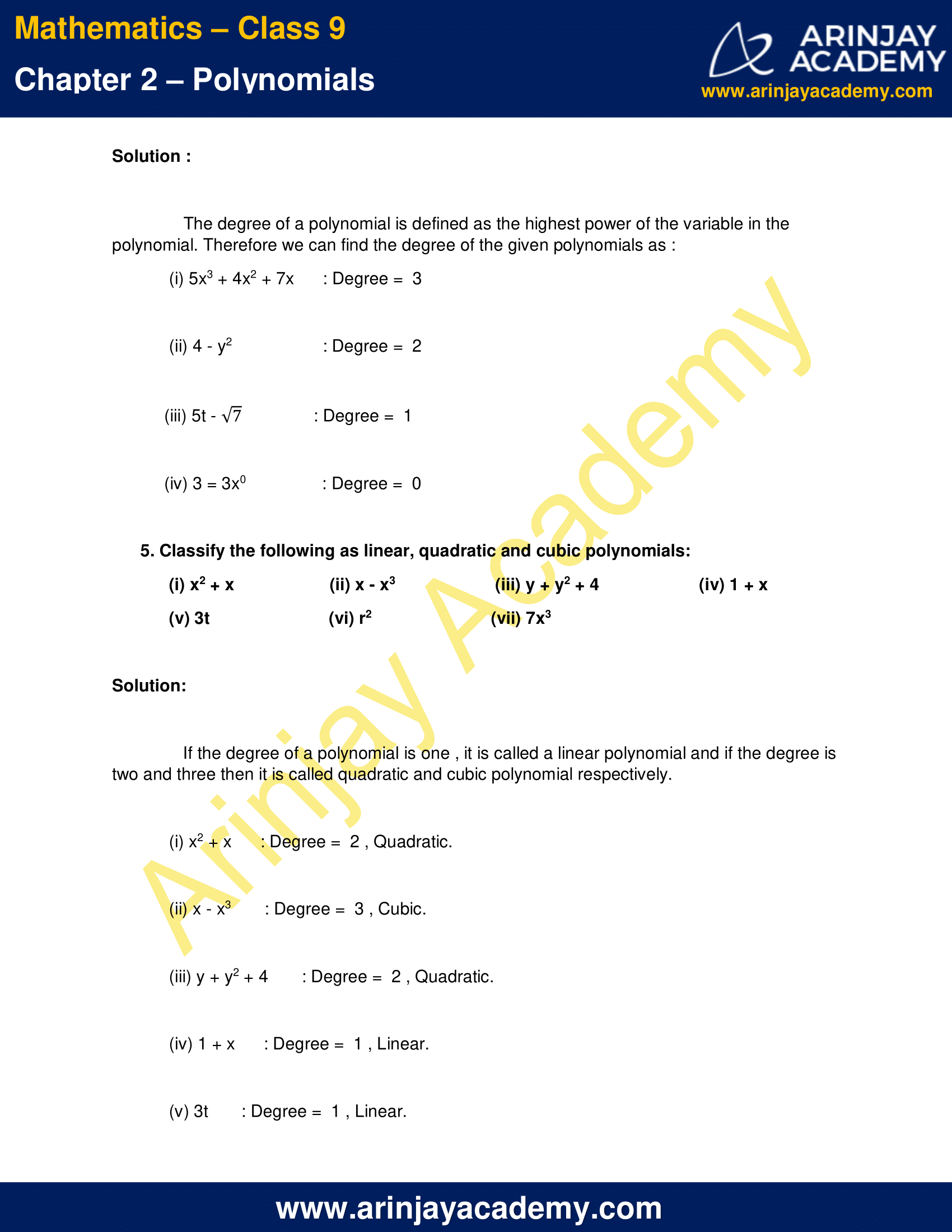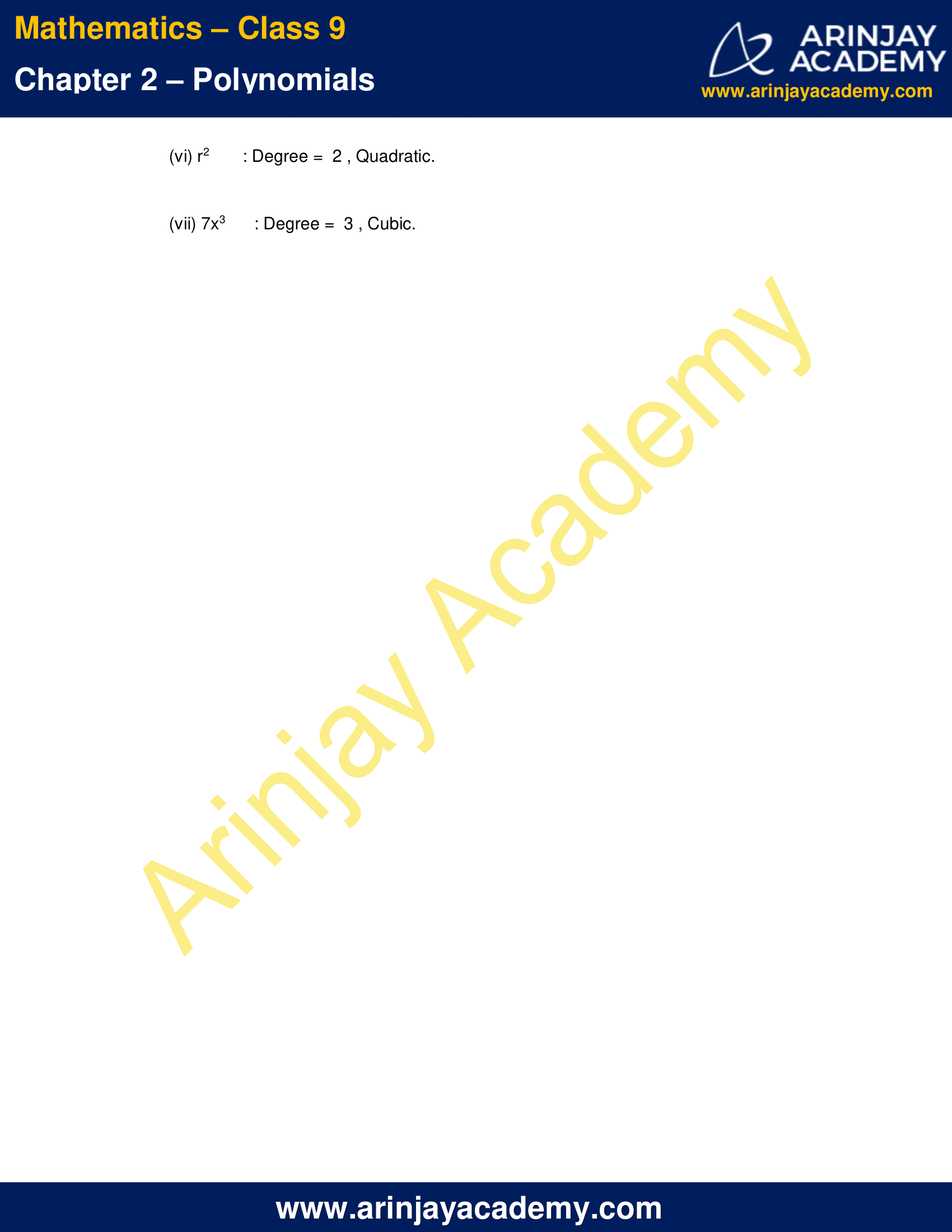# NCERT Solutions For Class 9 Maths Chapter 2 Exercise 2.1 – Polynomials

Download NCERT Solutions For Class 9 Maths Chapter 2 Exercise 2.1 – Polynomials. This Exercise contains 5 questions, for which detailed answers have been provided in this note. In case you are looking at studying the remaining Exercise for Class 9 for Maths NCERT solutions for Chapter 2 or other Chapters, you can click the link at the end of this Note.

### NCERT Solutions For Class 9 Maths Chapter 2 Exercise 2.1 – PolynomialsNCERT Solutions For Class 9 Maths Chapter 2 Exercise 2.1 – Polynomials

1. Which of the following expressions are polynomials in one variable and which are not? State reasons for your answer.

(i) 4x2 – 3x + 7
(ii) y2
+  √2
(iii) 3√t + t√2
(iv) y + 2/y
(v) x10 + y3 + t50

Solution:

(i) 4x2 – 3x + 7 : Exponent of each variable in the expression is a whole number and has one variable i.e. x . Therefore it is polynomial in one variable.

(ii) y2 +  √2  : Exponent of each variable in the expression is a whole number and has one variable i.e. y . Therefore it is polynomial in one variable.

(iii) 3√t + t√2  : Exponent of t in the term √t  is 1/2 i.e. not a whole number . Therefore it is not a polynomial.

(iv) y + 2/y : Exponent of y in the term 2/y is -1 i.e. not a whole number . Therefore it is not a polynomial.

(v) x10 + y3 + t50 : There is more than one variable in the expression . Therefore it is not a polynomial in one variable.

2. Write the coefficients of x2 in each of the following:

(i) 2 + x2 + x
(ii) 2 – x2 + x3
(iii) (Π/2)x2
+ x
(iv) √2x − 1

Solution :

(i) 2 + x2 + x  :    Coefficients of x2   = 1

(ii) 2 – x2 + x3 :    Coefficients of x2   = -1

(iii) (Π/2)x2 + x : Coefficients of x2 = (Π/2)

(iv) √2x − 1

The given expression can be written as

0x2 + √2x − 1 : Coefficients of x2   = 0.

3. Give one example each of a binomial of degree 35, and of a monomial of degree 100.

Solution :

A binomial of degree 35 must contain two terms where the highest exponent of the variable should be 35.
For example =  x35 + 5

A monomial of degree 100 must contain one term where the exponent of the variable should be 100.
For example = x100

4. Write the degree of each of the following polynomials:

(i) 5x3 + 4x2 + 7x
(ii) 4 – y2
(iii) 5t – √7
(iv) 3

Solution :

The degree of a polynomial is defined as the highest power of the variable in the polynomial. Therefore we can find the degree of the given polynomials as :

(i) 5x3 + 4x2 + 7x : Degree =  3

(ii) 4 – y2 : Degree =  2

(iii) 5t – √7 : Degree =  1

(iv) 3 = 3x0 : Degree =  0

5. Classify the following as linear, quadratic and cubic polynomials:

(i) x2 + x
(ii) x – x3
(iii) y + y2 + 4
(iv) 1 + x
(v) 3t
(vi) r2
(vii) 7x3

Solution:

If the degree of a polynomial is one , it is called a linear polynomial and if the degree is two and three then it is called quadratic and cubic polynomial respectively.

(i) x2 + x : Degree =  2 , Quadratic.

(ii) x – x3 : Degree =  3 , Cubic.

(iii) y + y2 + 4 : Degree =  2 , Quadratic.

(iv) 1 + x : Degree =  1 , Linear.

(v) 3t : Degree =  1 , Linear.

(vi) r2 : Degree =  2 , Quadratic.

(vii) 7x3 : Degree =  3 , Cubic.

NCERT Solutions for Class 9 Maths Chapter 2 Exercise 2.1 – Polynomials, has been designed by the NCERT to test the knowledge of the student on the topic – Polynomials in One Variable

The next Exercise for NCERT Solutions for Class 9 Maths Chapter 2 Exercise 2.2 – Number System can be accessed by clicking here.

Download NCERT Solutions For Class 9 Maths Chapter 2 Exercise 2.1 – Polynomials